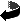# Mass endomorphism and spinorial Yamabe type problems on conformally flat manifolds by Bernd Ammann, Emmanuel Humbert, Bertrand Morel

Mass endomorphism and spinorial Yamabe type problems on conformally flat manifolds (.dvi, .ps,.ps.gz oder .pdf)
Comm. Anal. Geom. 14 no. 1, 163-182 (2006)

### Abstract

Let $M$ be a compact manifold equipped with a Riemannian metric $g$ and a spin structure $\si$. We let $\lamin (M,[g],\si)= \inf_{\tilde{g} \in [g] } \lambda_1^+(\tilde{g}) Vol(M,\tilde{g})^{1/n}$ where $\lambda_1^+(\tilde{g})$ is the smallest positive eigenvalue of the Dirac operator $D$ in the metric $\tilde{g}$. A previous result stated that $\lamin(M,[g],\si) \leq \lamin(\mS^n) =\frac{n}{2} \om_n^{{1/n}}$ where $\om_n$ stands for the volume of the standard $n$-sphere. In this paper, we study this problem for conformally flat manifolds of dimension $n \geq 2$ such that $D$ is invertible. E.g. we show that strict inequality holds in dimension $n\equiv 0,1,2\mod 4$ if a certain endomorphism does not vanish. Because of its tight relations to the ADM mass in General Relativity, the endomorphism will be called mass endomorphism. We apply the strict inequality to spin-conformal spectral theory and show that the smallest positive Dirac eigenvalue attains its infimum inside the enlarged volume-$1$-conformal class of $g$.

### Mathematics Subject Classification

53 A 30, 53C27 (Primary), 58 J 50, 58C40 (Secondary)Zurück zur Homepage

The Paper was written on 10.12.2003
Last update 11.12.2003